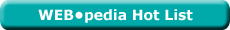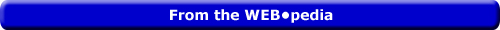Sunday  February 5, 2023
 AmosWEB means Economics with a Touch of Whimsy!FACTOR DEMAND AND MARGINAL REVENUE PRODUCT: For a firm that hires the services of a factor in a perfectly competitive factor market, the factor demand curve is that portion of the marginal revenue product curve that lies below the average revenue product curve. The relation between marginal revenue product and factor demand for a perfectly competitive firm is comparable to the relation between marginal cost and short-run supply. A perfectly competitive firm maximizes profit by hiring the quantity of a factor that equates factor price and marginal revenue product. As such, the firm moves along it's marginal revenue product curve in response to alternative factor prices.Most Viewed (Number) production stages (942)long-run production analysis (323)limited resources (216)foreign sector (212)unlimited wants and needs (211) Visit the WEB*pediaLesson Contents Unit 1: The Concept What It Is Circular Flow Unit 1 Summary Unit 2: Doing More Expenditures Consumption Expenditures Investment Expenditures Government Purchases Net Exports Unit 2 Summary Unit 3: The Curve Highlights Slope Real-Balance Effect Interest-Rate Effect Net-Export Effect Unit 3 Summary Unit 4: Determinants Instability Shifts: Increase Shifts: Decrease Unit 4 Summary Unit 5: Policies Plus Business Cycles Policies Unit 5 Summary Course Home
Aggregate Demand

This lesson introduces aggregate demand, the demand-side of the aggregate market. The aggregate market is the key model used to explain and analyze the workings of the macroeconomy and aggregate demand is a critical half of this model (the other is aggregate supply). Taking a clue from market demand, this lesson examines the nature of aggregate demand, including the relation between the price level and aggregate expenditures, the reason the aggregate demand curve is negatively sloped, and the assorted aggregate demand determinants that cause the aggregate demand curve to shift.

• The first unit of this lesson introduces the concept of aggregate demand and how it fits into the study of macroeconomics in terms of the aggregate market and circular flow.
• In the second unit, we example the four aggregate expenditures -- consumption, investment, government purchases, and net exports -- the make up aggregate demand.
• The third unit then examines the aggregate demand curve that captures the aggregate demand relation between the price level and aggregate expenditures, especially the importance of the real-balance, interest-rate, and net-export effects.
• A look at the assorted aggregate demand determinants that shift the aggregate demand curves is the topic of the fourth lesson.
• We end this lesson in the fifth unit with a look how demand-management policies work to stabilize business cycles through aggregate demand.

|ARC ELASTICITY

The average elasticity for discrete changes in two variables. The distinguishing characteristic of arc elasticity is that percentage changes are calculated based on the average of initial and ending values of each variable, rather than initial values. Arc elasticity is generally calculated using the midpoint elasticity formula. The contrast to arc elasticity is point elasticity. For infinitesimally small changes in two variables, arc elasticity is the same as point elasticity.WHITE GULLIBON[What's This?] Today, you are likely to spend a great deal of time watching infomercials trying to buy either a weathervane with a horse on top or a case of blank recordable DVDs. Be on the lookout for slow moving vehicles with darkened windows.Your Complete ScopeNatural gas has no odor. The smell is added artificially so that leaks can be detected."Live in such a way that you would not be ashamed to sell your parrot to the town gossip."-- Will RogersWPIWholesale Price IndexA PEDestrian's Guide Xtra CreditTell us what you think about AmosWEB. Like what you see? Have suggestions for improvements? Let us know. Click the User Feedback link.| | | | | | | | | | |
| | | |

Thanks for visiting AmosWEB